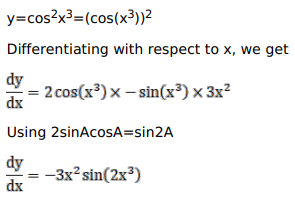# Solve this following

Question:

Mark $(\sqrt{)}$ against the correct answer in the following:

If $y=\cos ^{2} x^{3}$ then $\frac{d y}{d x}=$ ?

A. $-3 x^{2} \sin$

B. $-3 x^{2} \sin ^{2} x^{3}$

C. $-3 x^{2} \cos ^{2}\left(2 x^{3}\right)$

D. none of these

Solution: